# Challenging Maths Subtraction Questions for KidsPresenting simple easy-to-solve subtraction questions for kids. Before we explore about subtraction sums for Class 1, we need to incorporate an understanding of subtraction into the young minds. Subtraction is one of the core functions of Arithmetic. It is the difference in the final result by deducting one number from another. Presented here are some exciting questions for kids with answers.

Kids who have just stepped into school tend to learn the basic mathematical concepts like subtraction sums of one-digit Maths facts, double-digit Maths subtraction questions with numbers from 0-10, 0-20 and 0-30, practice subtraction problems with whole numbers from 0 to 10. In the mathematical world, subtraction is often referred to as a minus (-). You can download the simple subtraction worksheets for Grade 1 PDF from the download link given below.

Here, we bring to you a few simple subtraction questions with answers that children can find useful while studying Maths:

Single-digit subtraction: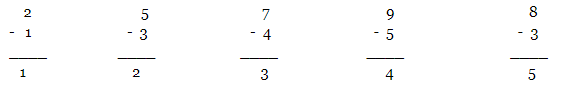Subtraction of a single-digit number form a double-digit number:

Steps to solve the subtraction of a single-digit number from a double-digit number.

Example: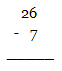Step 1: Subtract the ones place digits (6 – 7). Since it is not possible, borrow “1” from the tens place. The ones place digit becomes 16 and now subtract i.e. 16 – 7 = 9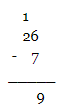Step 2: Subtract the tens place digits i.e. 1(1 borrowed) – 0 = 1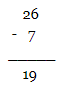Some more subtraction sums:-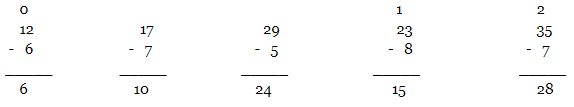Subtraction of a double-digit number from a double-digit number: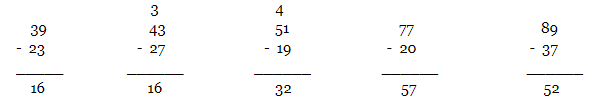Subtraction of a double-digit number from a triple-digit number: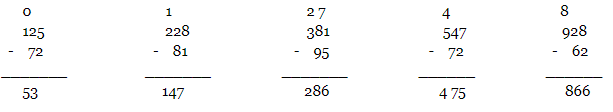Subtraction of a triple-digit number from a triple-digit number:

Example: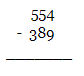Step 1: Subtract the ones place digits (4 – 9). Since it is not possible, borrow “1” from the tens place. The ones place digit becomes 14 and now subtract i.e. 14 – 9 = 5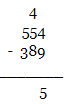Step 2: Subtract the tens place digits i.e. 4(1 borrowed) – 8. Since it is not possible, borrow “1” from the hundreds place. The tens place digit becomes 14 and now subtract i.e. 14 – 8 = 6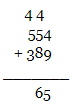Step 3: Subtract the hundreds place digits i.e. 4(1 borrowed) – 3 = 1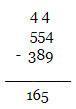Some more subtraction sums:-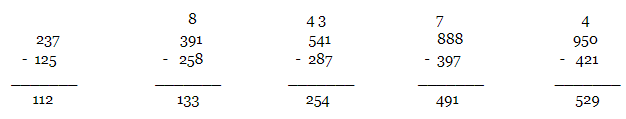Kids learn to solve simple subtraction problems from a very young age. These subtraction questions are included in the subtraction worksheets for Grade 1 PDF given above that involves finding the difference between single or double-digit numbers, column Maths subtraction questions, etc. We also offer question sheets on various other topics which you might want to check out.

You can also visit our Kids Learning section which is full of fascinating learning resources for children. You will find numerous engrossing worksheets, brain-tickling general knowledge questions, trivia questions on various subjects, poems for kids, stories of all genres with colourful PDFs for free, NCERT solutions, and a lot more! Also, stay tuned to the BYJU’S website to explore a huge assemblage of kids learning resources with colourful pictures to make your child learn in a joyful manner.